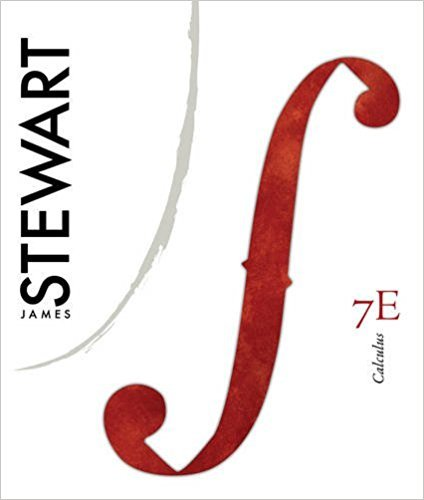×
×

# Solved: Make a rough sketch of a contour map for theISBN: 9780538497817 125

## Solution for problem 7 Chapter 14

Calculus, | 7th Edition

• Textbook Solutions
• 2901 Step-by-step solutions solved by professors and subject experts
• Get 24/7 help from StudySoup virtual teaching assistantsCalculus, | 7th Edition

4 5 1 251 Reviews
12
2
Problem 7

Make a rough sketch of a contour map for the function whose graph is shown

Step-by-Step Solution:
Step 1 of 3
Step 2 of 3

Step 3 of 3

##### ISBN: 9780538497817

The full step-by-step solution to problem: 7 from chapter: 14 was answered by , our top Calculus solution expert on 11/10/17, 05:23PM. This full solution covers the following key subjects: contour, function, graph, make, Map. This expansive textbook survival guide covers 17 chapters, and 887 solutions. The answer to “Make a rough sketch of a contour map for the function whose graph is shown” is broken down into a number of easy to follow steps, and 15 words. Since the solution to 7 from 14 chapter was answered, more than 234 students have viewed the full step-by-step answer. This textbook survival guide was created for the textbook: Calculus,, edition: 7. Calculus, was written by and is associated to the ISBN: 9780538497817.

Unlock Textbook Solution